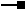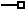This document is archived and information here might be outdated.  Recommended version.

IGeometryDefEdit Interface (ArcObjects .NET 10.8 SDK)
 ArcObjects Help for .NET developers > ArcObjects Help for .NET developers > ArcObjects namespaces > Geodatabase > ESRI.ArcGIS.GeoDatabase > Interfaces > IG > IGeometryDefEdit Interface
 ArcGIS Developer Help

# IGeometryDefEdit Interface

#### Product Availability

Available with ArcGIS Engine, ArcGIS Desktop, and ArcGIS Server.

#### Members

Name DescriptionAvgNumPoints The estimated average number of points per feature.AvgNumPoints The estimated average number of points per feature.GeometryType The geometry type.GeometryType The enumerated geometry type.GridCount The number of spatial index grids.GridCount The number of spatial index grids.GridSize The size of a spatial index grid.GridSize The size of a spatial index grid.HasM Indicates if the feature class will support M values.HasM Indicates if the feature class has measure (M) values.HasZ Indicates if the feature class will support Z values.HasZ Indicates if the featureClass has Z values.SpatialReference The spatial reference of the dataset.SpatialReference The spatial reference for the dataset.

#### Inherited Interfaces

Interfaces Description

#### Classes that implement IGeometryDefEdit

Classes Description
GeometryDef Esri Geometry Definition object.

#### Remarks

The IGeometryDefEdit interface is used when creating a GeometryDef object. You would normally use this interface when defining a new feature class. You cannot use IGeometryDefEdit to modify an existing GeometryDef which is attached to a feature class; the same restrictions apply when using the standard ArcGIS user interface. For example, you cannot change the spatial reference of an existing feature class.

The following are valid Geometry types for a new feature class (from esriGeometryType):

esriGeometryPoint

esriGeometryMultipoint

esriGeometryPolyline

esriGeometryPolygon

esriGeometryMultiPatch

The SpatialReference property should always be set when creating a new GeometryDef.  If a GeometryDef without a spatial reference is used to create a stand-alone feature class, an error will occur.

If a feature class is created in a feature dataset, and the SpatialReference property does not match the spatial reference of the feature dataset, the property will be modified to match that of the feature dataset.

[C#]

The code below shows how to create a geometry field, assuming a spatial reference has already been created:

// Create the GeometryDef and set its spatial reference and geometry type.
IGeometryDef geometryDef = new GeometryDefClass();
IGeometryDefEdit geometryDefEdit = (IGeometryDefEdit)geometryDef;
geometryDefEdit.SpatialReference_2 = spatialReference;
geometryDefEdit.GeometryType_2 = esriGeometryType.esriGeometryPolyline;

// Set the grid count to 1 and the grid size to 0 to allow ArcGIS to
// determine a valid grid size.
geometryDefEdit.GridCount_2 = 1;
geometryDefEdit.set_GridSize(0, 0);

// Create a geometry field and assign the GeometryDef to it.
IField field = new FieldClass();
IFieldEdit fieldEdit = (IFieldEdit)field;
fieldEdit.Name_2 = "SHAPE";
fieldEdit.Type_2 = esriFieldType.esriFieldTypeGeometry;
fieldEdit.GeometryDef_2 = geometryDef;

[Visual Basic .NET]

The code below shows how to create a geometry field, assuming a spatial reference has already been created:

' Create the GeometryDef and set its spatial reference and geometry type.
Dim geometryDef As IGeometryDef = New GeometryDefClass()
Dim geometryDefEdit As IGeometryDefEdit = CType(geometryDef, IGeometryDefEdit)
geometryDefEdit.SpatialReference_2 = spatialReference
geometryDefEdit.GeometryType_2 = esriGeometryType.esriGeometryPolyline

' Set the grid count to 1 and the grid size to 0 to allow ArcGIS to
' determine a valid grid size.
geometryDefEdit.GridCount_2 = 1
geometryDefEdit.GridSize_2(0) = 0

' Create a geometry field and assign the GeometryDef to it.
Dim field As IField = New FieldClass()
Dim fieldEdit As IFieldEdit = CType(field, IFieldEdit)
fieldEdit.Name_2 = "SHAPE"
fieldEdit.Type_2 = esriFieldType.esriFieldTypeGeometry
fieldEdit.GeometryDef_2 = geometryDef